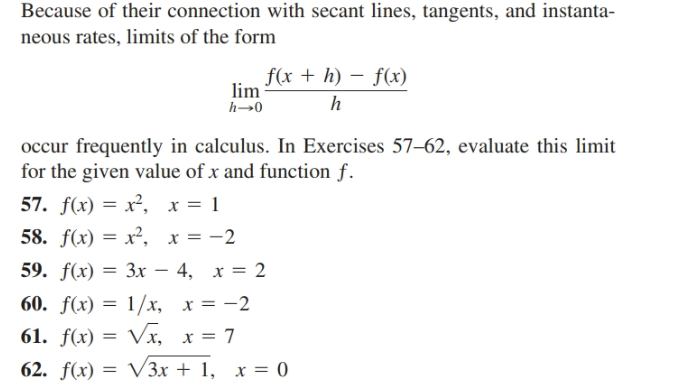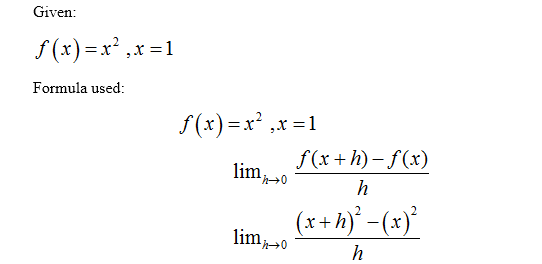# Because of their connection with secant lines, tangents, and instanta-neous rates, limits of the formf(x + h) – f(x)limoccur frequently in calculus. In Exercises 57–62, evaluate this limitfor the given value of x and function f.57. f(x) = x², x = 158. f(x) = x², x = -259. f(x) — Зх — 4, х %3D 260. f(x) = 1/x, x = -261. f(x) = Vx, x = 762. f(x) = V3x + 1, x = 0

Question
1 viewshelp_outlineImage TranscriptioncloseBecause of their connection with secant lines, tangents, and instanta- neous rates, limits of the form f(x + h) – f(x) lim occur frequently in calculus. In Exercises 57–62, evaluate this limit for the given value of x and function f. 57. f(x) = x², x = 1 58. f(x) = x², x = -2 59. f(x) — Зх — 4, х %3D 2 60. f(x) = 1/x, x = -2 61. f(x) = Vx, x = 7 62. f(x) = V3x + 1, x = 0 fullscreen
check_circle

Step 1

We’ll answer the first question since the exact one wasn’t specified. Please submit a new question specifying the one you’d like answered....

### Want to see the full answer?

See Solution

#### Want to see this answer and more?

Solutions are written by subject experts who are available 24/7. Questions are typically answered within 1 hour.*

See Solution
*Response times may vary by subject and question.
Tagged in